## Monday, 28 November 2016

### Deconstructing Construction Vibrations

Ground vibrations can come from a large number of different sources, such as rail and vehicular traffic, jackhammering, bulldozing, pavement milling, vibratory compaction, pile driving, and blasting.

The magnitude of these vibrations can vary from being imperceptible, to causing a minor nuisance, to possibly even causing extreme damage. Generally speaking, the main factors to consider are the strength of the source vibrations, duration of exposure, distance from the source, type and condition of the affected building, and the local soil type. Our building stock and infrastructure are aging. More and more there will be a need to carry out construction activities near these aging buildings. Consequently, there will likely be a rise in the number of complaints, and insurance claims, due to construction-induced ground vibrations in the future.

Ground vibrations are typically quantified in terms of Peak Particle Velocity (PPV), usually expressed in millimetres per second (mm/s) or inches per second (in/sec). Stronger vibrations have a higher PPV. Vibrations can damage buildings directly as a result of the movement in the walls or floors, or the damage can be indirect as a result of soil movements that were caused by the vibrations. In Canada, there is no national standard for ground vibrations, but standards from other countries generally agree that ground vibrations should be limited to 5 mm/s to prevent direct damage to small residential buildings. This 5 mm/s threshold is based on empirical data and probabilities. The threshold is intended to ensure there is a very low risk of cosmetic cracking in drywall, plaster, and old unreinforced masonry. There is no guarantee that damage cannot occur at lower levels, but that would be very rare. There's also no guarantee that exceeding the 5 mm/s threshold will cause damage to a building. In fact, most buildings can tolerate stronger vibrations, especially buildings that are well-constructed, in good condition, and/or are only briefly exposed to the vibration.

Whether or not an activity will exceed a vibration threshold depends on the distance from the source, local soils, and the strength of the source vibrations. Weak and soft soils are good at dissipating vibrations, while hard soils or rock can transmit vibrations a long way. Just think of how you would feel the vibrations in your hands if you swung a steel pipe at concrete wall as hard as you could, and how different your hands would feel if you swung a pillow instead. But remember, the 5 mm/s threshold is for direct damage to the building, so it might not be applicable if the building sits on a soil that is susceptible to vibrations, making the building vulnerable to indirect damage.

Susceptibility to indirect damage depends on the local soil and the duration of exposure to vibrations. Susceptible soils include sand, gravel, and some fine-grained soils known as loess. These soils can be consolidated by vibrations, which can in turn result in foundation movement and cracking. Imagine the particles in a loosely compacted sand are like a house of cards. The vibration makes the particles move around and fall into a more compact configuration, like when a house of cards collapses. The amount of consolidation that can take place depends on how well these soils were compacted beforehand and how long they're exposed to the vibrations. There are no standards limiting vibrations to prevent soil consolidation because more research into the problem is needed. However, most vibration standards at least recognize that problems can occur with loose granular soils and recommend that a geotechnical engineer be consulted. Limited available empirical data on damage caused by vibration-induced soil consolidation shows that some buildings in loess soils have been damaged at vibration levels below 1 mm/s. Therefore, both the soil characteristics and the ground vibrations need to be considered together when investigating vibration damage.

Building occupants are typically much more sensitive to vibrations than the structures themselves. Vibrations of just 0.3 mm/s could be sufficient to disturb some homeowners and vibrations of about 1 mm/s are likely to cause complaints. A vibratory roller compactor working in an area with hard soils might cause complaints more than 400 feet away! Therefore, the sensitivity of the occupants is frequently an issue with vibration damage claims. Often, when building owners perceive nuisance vibrations they go looking for damage afterwards. They then find a number of cracks that they hadn't ever paid any attention to before. In many cases, most or all of the damage reported was actually pre-existing.

Cracking caused by ground vibrations may be indistinguishable from other common types of damage, like cracking from thermal and moisture movements or small foundation settlements. Therefore, a vibration damage investigation involves a lot more than a visual examination. Additional information needs to be considered, including the local soil conditions, type of building involved, general condition of the building, and the duration, frequency, and source location of the vibrations. Documentation of pre-existing conditions can be the most valuable information for an investigation.

If you look hard enough, you'll find cracks in any house. If you're a homeowner, it's a good idea to looking for cracks once in a while and document them with photos. You should also do this as soon as you see any major construction work going on nearby. That way, if damage does occur, you will have some documentation of existing conditions from when the construction work started. That record of pre-existing conditions will help in determining the extent of the damages.

If you're a contractor, the best way to prevent complaints is to inform nearby property owners about the ground vibrations they can expect and to conduct pre-construction surveys to document any existing cracks. Nuisance vibrations are more tolerable when they are expected, and if you do the pre-construction survey then owners won't be discovering pre-existing cracks while the construction work is going on. And if damage does occur, that record of pre-existing conditions will help limit the costs to just the vibration damage.

References
Dowding, C.H. (2000). Construction Vibrations. Second edition. Prentice Hall, Upper Saddle River, NJ.

Svinkin, M.R. (2012). "The necessity of condition surveys for structural protection against pile driving effects," Proceedings of the 9th International Conference on Testing and Design Methods for Deep Foundations, T. Matsumoto, ed., Kanazawa e-Publishing, Japan, 429-439.

Svinkin, M.R. (2015). "Tolerable Limits of Construction Vibrations," Practice Periodical on Structural Design and Construction. 10.1061/(ASCE)SC.1943-5576.0000223.

Wiss, J.F. and Parmalee, R.A. (1974). "Human Perception of Transient Vibrations," Journal of the Structural Division, ASCE, Vol. 100, No. S74, pp. 773-787.

## Thursday, 29 September 2016

### For a Few Birthdays MoreOkay, maybe it CAN be worse than Paint Your Wagon.

It's that time again. Time to solve some math problem involving birthdays. I've previously solved the Classic Birthday Problem, as well as the Near-Match, First Match (and First Near-Match), Same Birthday as You, and Matched Couples (and larger groupings) variations.

I'm going to call this the Cover All Birthdays variation. We're looking for the probability that a group of people has every day of the year covered for birthdays.

Let's start by looking at a simpler problem. Suppose that we're tossing rings at some pegs. In this scenario we're assuming that every peg has an equal probability of being hit and that every ring must land on a peg. For any given numbers of n rings and k pegs, what's the probability that every peg is hit at least once? It is impossible to hit k pegs with fewer than k rings, so there is an implicit assumption in this analysis that n k.

With only one peg, the problem is trivial because all rings necessarily land on that one peg. There is only one possible outcome irrespective of n: the probability of hitting all pegs is 100%.

Since rings can land on any peg, including on the same peg multiple times, the total number of possible outcomes in this ring toss scenario is equal to k to the n-th power. To calculate the probability of hitting all pegs we need to count all of the ways that we could have at least one lone peg. So let's look at a two-peg situation now. There are 2 to the n-th power possible outcomes with the ring tosses. If we have one lone peg, that means there's just one peg left to hit. We already know that there's only one way to hit just one peg. But now, either peg could be the one that gets all the rings, so with 2 pegs there are 2*1 = 2 possible ways to have a lone peg. That means there are 2^n - 2 ways of hitting each peg at least once. The probability of covering all the pegs is therefore [2^n - 2] / [2^n] = 1 - 2^(1-n).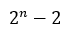Number of ways to throw n rings at 2 pegs, hitting all pegs at least once.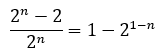Probability of hitting all pegs at least once when throwing n rings at 2 pegs.

What happens when we add in a third peg? Again, we could have all the rings land on one peg. This time there are 3 different pegs that could get all the rings, so there are 3 * 1 = 3 ways to hit only one peg. We already figured out that there were 2^n - 2 ways of covering 2 pegs, but now there are three pegs we could choose the two from, or C(3,2) = 3 ways to pick two out of three pegs. That gives us a total of 3 * (2^n - 2) ways of covering exactly two out of three pegs. We're left with 3^n - 3*(2^n-2) - 3 ways of covering all the pegs, out of a total 3^n permutations. Therefore, the probability of covering all three pegs is: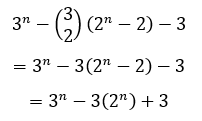Number of ways to throw n rings at 3 pegs, hitting all pegs at least once.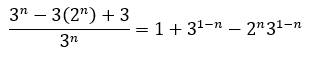Probability of hitting all pegs at least once when throwing n rings at 3 pegs.

Let's now define a function F[n,k] as the number of ways of hitting each of the k pegs at least once from n tosses. That recursive relationship looks like this:Recursive relationship defining the number of ways you can throw n rings hitting all k pegs at least once.Probability of throwing n rings and hitting all k pegs at least once.

As you keep adding pegs you'll see that the equations quickly start to get lengthy. Using the recursive relationship we could take a brute force calculation approach to evaluate F[n,k], but that quickly gets to be very inefficient as k increases. We'd like a different form of the equation. If we expand and simplify for k = 4 and k = 5, we get the following: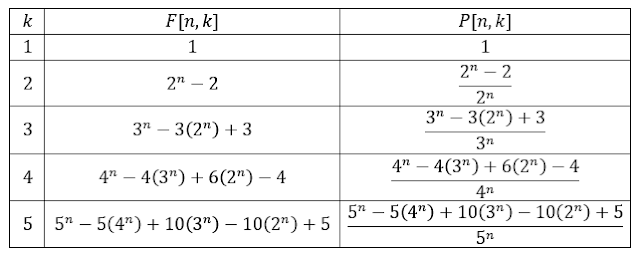Expanded and simplified expressions for F[n,k] and P[n,k] for k = 1 to 5.

Just for fun, let's plot those out as well so you can see how many tosses you need to have a reasonable chance of hitting all of the pegs.Tabulated values of the probability of throwing n rings and hitting all k pegs at least onceGraph showing the probability of hitting all pegs at least once with n tosses.

In order to have at least 50% chance of hitting all of the pegs, you need only 2 tosses when there are 2 pegs, 5 tosses for 3 pegs, 7 tosses for 4 pegs, and 10 tosses for 5 pegs.

Now, going back to the expanded equations we wrote out, you might've spotted a pattern. We can define F[n,k] in closed-form using binomial coefficients and summation notation as follows:Solution to the recursive relation for F[n,k].

Okay, so how does this relate to the Cover All Birthdays problem? Well if instead of n rings we have n randomly selected people and instead of k pegs we have 365 possible days a person could be born on, the problem is modeled exactly the same way. There are 365^n permutations of birthdays in a group of n people and there are F[n,365] ways of covering each possibility at least once:Number of birthday combinations in a group of n people where every day of the year is covered at least once.

Dividing by 365^n to get the probability of covering all birthdays, we can make the following graph:Probability of covering all 365 birthdays in a group of randomly selected people.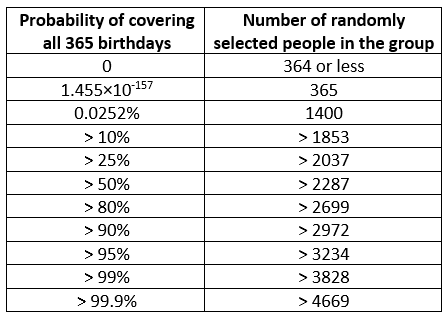Size of group required to achieve the given threshold probabilities of covering all 365 birthdays.

For n < 365 we know the probability of covering all the birthdays is exactly zero. For n = 365, there's less than 1 in 6.873×10¹⁵⁶ chance of covering every day of the year. If we double that to n = 730, we increase the probability by a factor of nearly 3.45×10¹²⁸, but that's still a negligibly small chance of about 1 in 1.993×10²⁸. Chances of covering all birthdays are vanishingly small until approximately n = 1400. We need at least n = 2287 to exceed a 50% chance of covering all birthdays and at least n = 3828 to give us better than a 99% chance.

## Friday, 16 September 2016

### Masonry Patterns

The simplest and most common patterns in modern masonry construction are running bond and stack bond. In running bond, masonry units in one course are centred over the head joints in the course below. As any child who’s experimented with blocks knows, this interlocking pattern helps distribute loads and makes the structure more robust. In stack bond, masonry units line up directly with those in the course below. The continuous head and bed joints give the masonry a distinct grid pattern that can have a pleasing aesthetic, particularly when the mortar joints are raked. However, masonry constructed in stack bond is weaker and less robust than masonry constructed in running bond. That said, a carefully designed structure that properly utilizes the modular dimensions of concrete blocks can potentially be less expensive to build in stack bond pattern than in running bond pattern because there’s no need to cut half-blocks at openings.
Figure 1: Running Bond (left) and Stack Bond (right).

Variations of running bond where the offset from one course to the next is somewhere between half a unit (ordinary running bond) and zero (stack bond) are also fairly common. While there could be an infinite number of possibilities here, the most common running bond variants are one-third running bond (1/3 offset) and one-quarter running bond (1/4 offset).

Figure 2: One-Third Running Bond (left) and One-Quarter Running Bond (right).

In addition to the patterns still in common use, there are dozens of other possible patterns; too many to discuss. Some of which were common in the past when multi-wythe construction was common, and some are rare patterns that were simply invented to create unique aesthetics. Below are just a few examples of the different patterns in existence: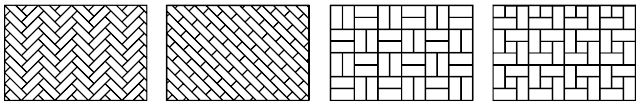Figure 3 (from left to right): 45° Herringbone Bond, Diagonal Bond, Double Basket Weave Bond, and Pinwheel Bond.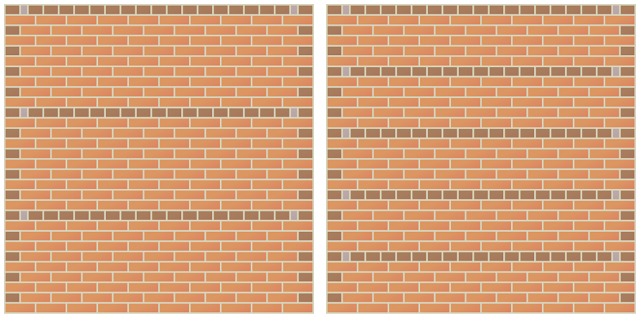Figure 4: American Bond (left) and Scottish Bond (right). Headers shown in brown, stretchers in orange, and queen closers in grey.Figure 5: English Bond (left) and Flemish Bond (right). Headers shown in brown, stretchers in orange, and queen closers in grey.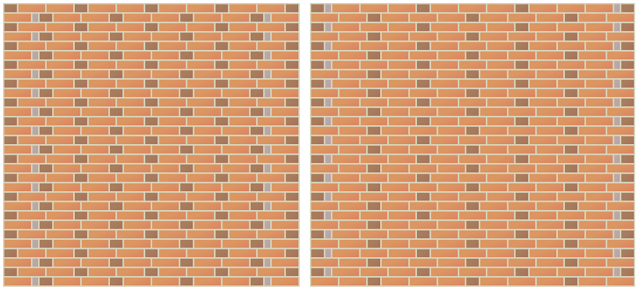Figure 6: Monk Bond (left) and Sussex Bond (right).Headers shown in brown, stretchers in orange, and queen closers in grey.

References
BIA. (1975). Technical Note 2: Glossary of Terms Relating to Brick Masonry. Brick Industry Association, Reston, VA.
BIA. (1999). Technical Note 30: Bonds and Patterns in Brickwork. Brick Industry Association, Reston, VA.
Brunskill, R. W. (1997). Brick Building in Britain. Gollancz, London, UK.
Hatzinikolas, M. A. and Korany, Y. (2005). Masonry Design for Engineers and Architects. Canadian Masonry Publications, Edmonton, Alberta.
Lloyd, N. (1925). A History of English Brickwork. Antique Collectors’ Club, Woodbridge, UK.

## Wednesday, 7 September 2016

### The Generalized Egg Drop Problem

The original puzzle goes something like this: You have some identical eggs and a 100-storey building. You do not know what is the highest floor one can drop an egg from without breaking it, but for some reason you need to find out. What is the minimum number of drops that would guarantee an answer for you if can use only two eggs for testing?

In order to be sure that you've found the maximum safe height you need to have tested the next floor above: the minimum height required to break an egg. If you had only one egg available for testing, you'd have no choice but to start at the first floor and move up one at a time until you broke the egg. But with a second egg, you can skip some floors, using the first egg to bracket the solution. We're trying to minimize the number of times we'd have to drop the second egg one floor at a time. Supposing that we have a maximum number of available drops, d, regardless of whether we break the eggs or not, then each time we drop the first egg without breaking it, that's one less drop available for the second egg. So the most efficient procedure is to skip to the dth floor first. If it doesn't break, you go up by another d-1 floors. It it still doesn't break, you go up d-2, d-3, etc. until it does break (or you run out of floors). When the first egg finally does break, you go back to the one floor above the last floor that didn't break the first egg and test the second egg. You keep going up one at a time until you break the second one (or it doesn't break on the floor below the one that broke the first egg, in which case you know that you stumbled upon the minimum height to break an egg using your first egg). Mathematically, the tallest building we can test with d drops and 2 available eggs is given by the summation: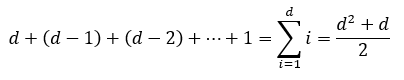So for the case of a building with 100 floors, we solve: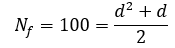This quadratic equation has two real roots, but we can ignore the negative root because it has no physical meaning for our problem. The positive real root is: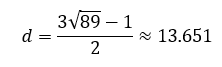A partial drop has no physical meaning either, so we have to round up to the next whole number, in this case 14. In other words, for a 100-floor building and 2 eggs, the minimum number of drops that will guarantee an answer is 14. You start by dropping the first egg on the 14th floor. If it doesn't break, you go up another 13 (to 27), then 12 (to 39), then 11 (to 50) etc. until it breaks, the test one floor at a time with the second egg.

Here are two examples of how the tests could go on our 100-storey building: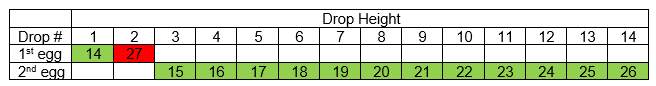Example of the optimal test procedure on a 100-floor building when only 2 eggs are available. Solution = 26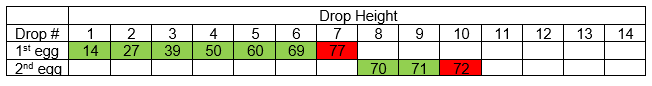Example of the optimal test procedure on a 100-floor building when only 2 eggs are available. Solution = 71

We can generalize the problem to any number of eggs, Ne. We don't know how many drops we will need, but if we specify a maximum of d drops, then hopefully we can solve for the maximum height, Nf of a building for which we are guaranteed be able to find a solution.

Now we don't know what Nf is yet, but let's pretend that we do already know how to find Nf for any numbers d drops and Ne eggs. We at least know that Nf is a function of d and Ne, which we can express mathematically as: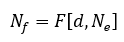What happens if we're told that we get one more egg and one more drop? Now the total number of floors we could test is F[d+1,Ne+1]. With our extra egg we'd like to bracket the solution such that if it breaks on our first drop we're left with only F[d,Ne] floors. So if we optimize our search procedure, when we're given one more egg and one more drop to start out with, there are two possible outcomes on the first drop:
1. We start out with F[d+1,Ne+1] floor to test. The first egg breaks on the first drop. We have Ne eggs and d drops remaining to find the maximum safe drop height from the remaining F[d,Ne] floors. We're pretending that we already know what the function F[d,Ne] is.
2. The first egg doesn't break on the first drop. Now we still have (Ne+1) eggs and d drops remaining for some number F[d,Ne+1] floors above.
This gives us a recursive relation that can be expressed mathematically as: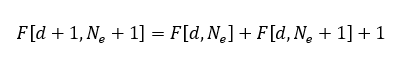or, equivalently: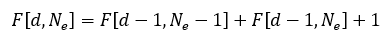Now as I said before, we still don't know what the general form of F[d,Ne] is, but we did already figured out the general form for when Ne = 2.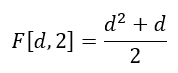We also know that when Ne = 1 we have no choice but to start at the bottom and go up one floor at a time until it breaks. The maximum number of floors we can test is equal to the number of times we can drop the egg.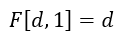There's also a simple boundary condition that will help us. If Ne = d, there can be no further benefit to adding another egg, because even if we broke an egg every time, we'd reach our limit on the number of drops first. This gives us a condition that can be expressed as: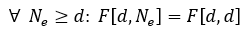We now have more than enough information to use the recursive relation and figure out how many floors could be tested with any number of eggs or drops. I've shown the first few steps below: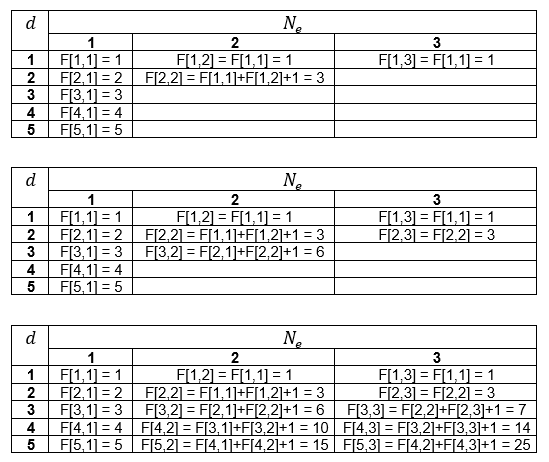Now if you use a spreadsheet it is fairly easy to automate these recursive calculations and create a large table of values, such as the one I made here: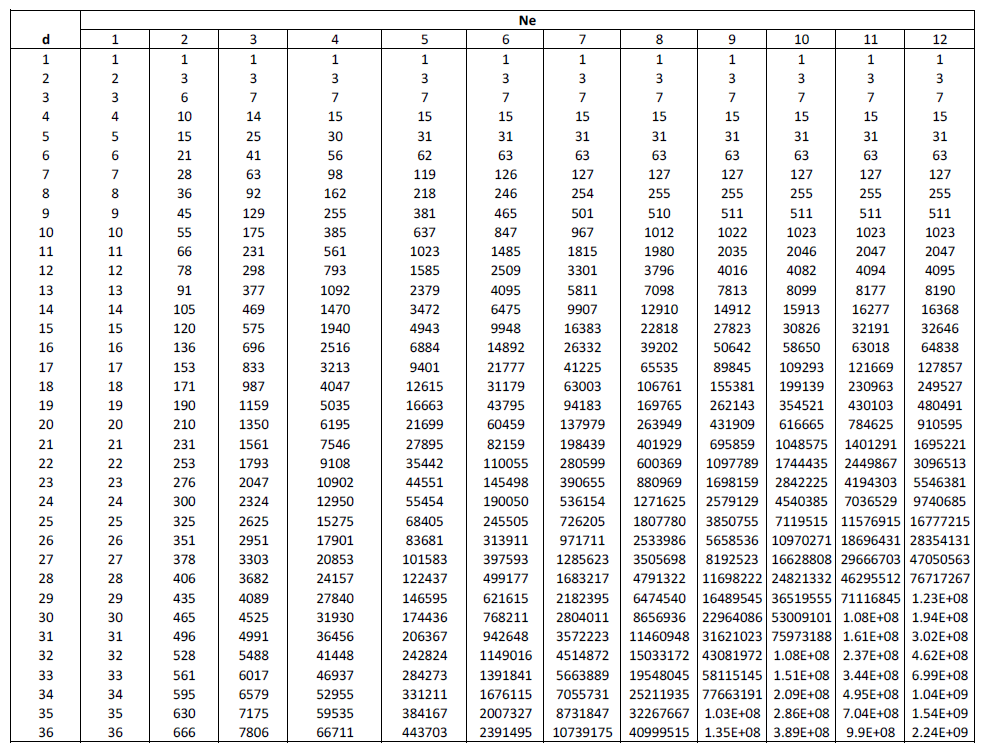Now we could stop here. Theoretically, we can produce a table of values of maximum building heights Nf for any numbers Ne eggs and d drops, though we have to do it by repeatedly adding some of our previous function evaluations together. If we already know the height of the building and the number of eggs available for testing, then we can go down the column for corresponding value of Ne until we reach the first value of Nf that is equal to or greater than the number of floors in our building. Then we go left across the row and read the value d. This will be the minimum number drops required to guarantee we find a solution to the maximum safe drop height in our building of Nf floors.

But, of course, we won't stop there. We still don't know how to express the function F[d,Ne], we just defined a recursive relation that allowed us to evaluate it anyway.

Using some optimal search algorithm there is one unique path to every possible solution within the building of Nf floors. If we have one egg, there are Nf = d paths, all of which involve breaking one egg. Each solution path represents a combination, a way to select 1 floor from d floors. One broken egg out of d floors. Using the binomial coefficient, we can express the number of ways of choosing 1 floor out of d floors as C(d,1) = d.

When we have two eggs, the maximum is (d)(d+1)/2 floors, or (d)(d+1)/2 unique solution paths. Only one egg breaks for some solution paths, while 2 eggs break for other solution paths. The total, (d)(d+1)/2, is equal to the sum of the one-egg paths plus the two-egg paths. Each one-egg path is a way of selecting one egg-breaking floor from d egg drops. C(d,1). Each two-egg path is a way of selecting two egg-breaking floors from d egg drops. C(d,2). So the total number of paths is: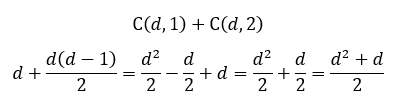Maybe you see where this is going. We haven't proven anything yet, but it looks like we can come up with a conjecture. Let us propose that for three eggs and d drops, the number of unique solution paths is equal to the number of paths where one egg breaks plus the number of paths where two eggs break plus the number of paths where three eggs break. In other words, C(d,1) + C(d,2) + C(d,3). Furthermore, let us propose that for Ne eggs and d drops, the number of unique solution paths is: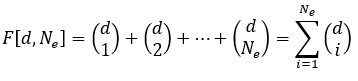Proposed general equation for the maximum number of floors that can be tested with d drops and Ne eggs.

Now all that's left to do is to prove the conjecture. I will use mathematical induction. We already know the conjecture is true for all d (greater than or equal to one) when Ne = 1 or 2. Next we assume that it is also true for any natural numbers d and Ne. If we can prove that the relation still holds true for d+1 and Ne+1, then the conjecture is true for all natural numbers. Starting with the recursive relation: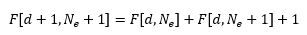Substituting the proposed general equation: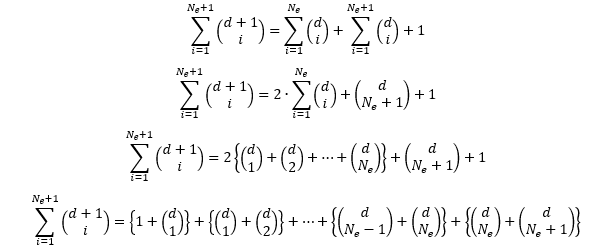Applying Pascal's Rule: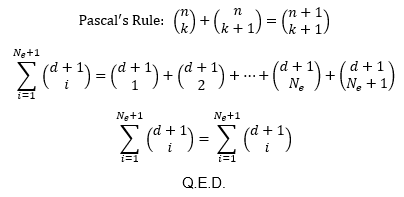So the conjecture has been proven. The maximum height of a building that can be checked using d drops and Ne eggs is Nf floors tall, where Nf is: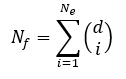Oh but we're still not done. The way the original problem was framed, we already know the height of the building and we're looking for the number of drops required. Unfortunately, there appears to be no general solution for that because it means having to solve for the positive real root of a polynomial with degree Ne. Apart from using a table as I described earlier, you could guess a value d, calculate Nf, and keep trying new guesses until you find the minimum value of d that gives Nf greater than or equal to the height of the building. But while we can't come up with a general solution for the minimum number of drops as a function of Nf and Ne, we can solve for a few cases. The cases Ne = 1 and Ne = 2 are easily done by hand. Closed-form solutions for the cubic and quartic functions exist, so the cases of Ne = 3 and Ne = 4 can also be solved analytically (though I definitely wouldn't attempt solving Ne = 4 by hand!). For Ne > 4, analytical solutions do not exist, with the exception of one special case. Here are the solutions for Ne = 1 to 4.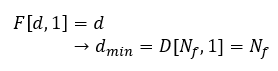Minimum number of drops when 1 egg is available for testing.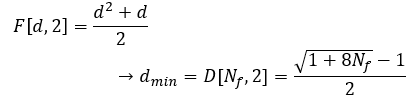Minimum number of drops when 2 eggs are available for testing.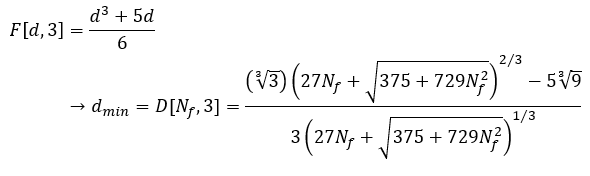Minimum number of drops when 3 eggs are available for testing.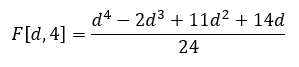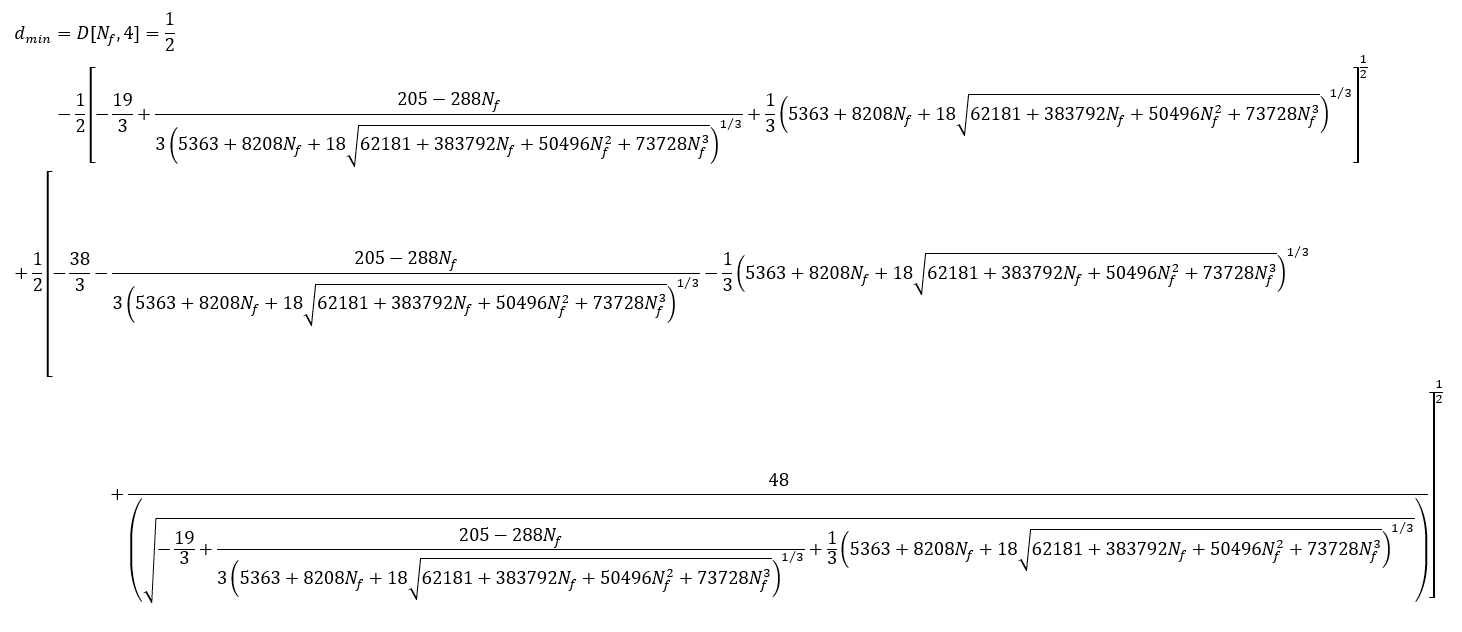Minimum number of drops when 4 eggs are available for testing.

Now I mentioned there was special case that could also be solved. That is the case of "many" eggs, that is, when Ne is at least equal to d. In this case: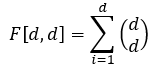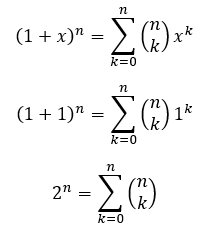And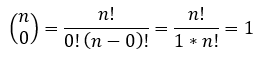Therefore,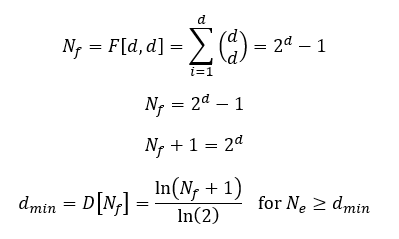Minimum number of drops when there are "many" eggs available for testing.

Finally. After all of that math, here's is a table and some graphs of the minimum number of drops required to find the maximum safe drop height in a building with Nf floors and with Ne eggs available for testing.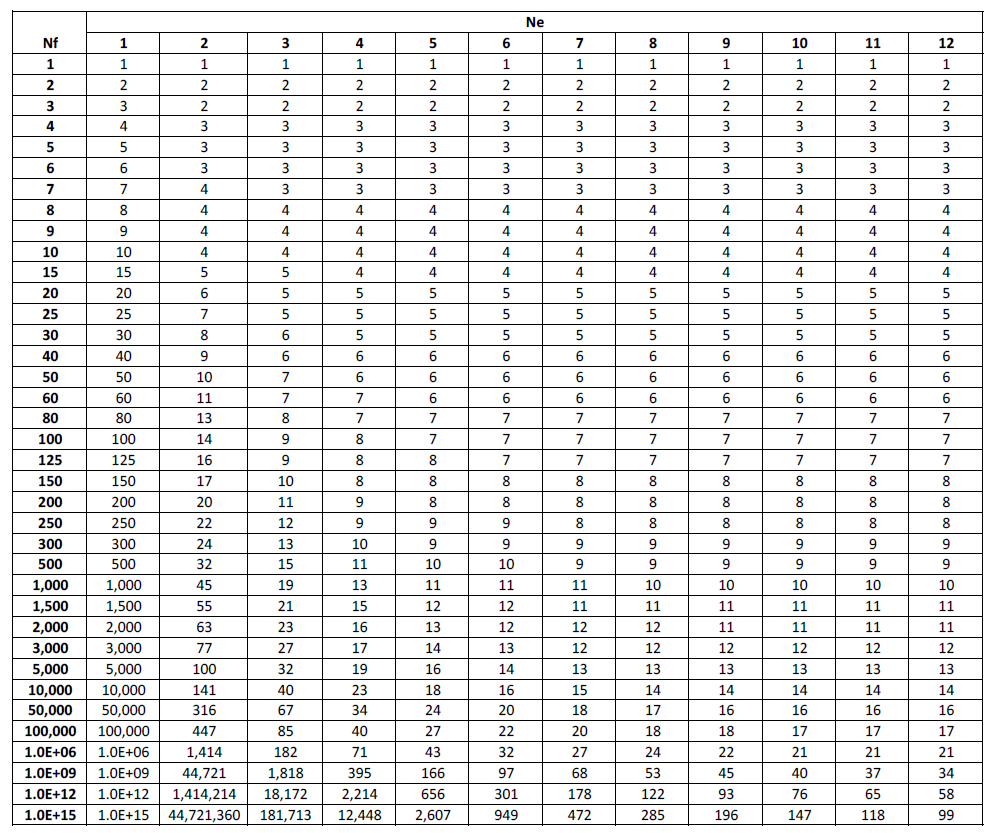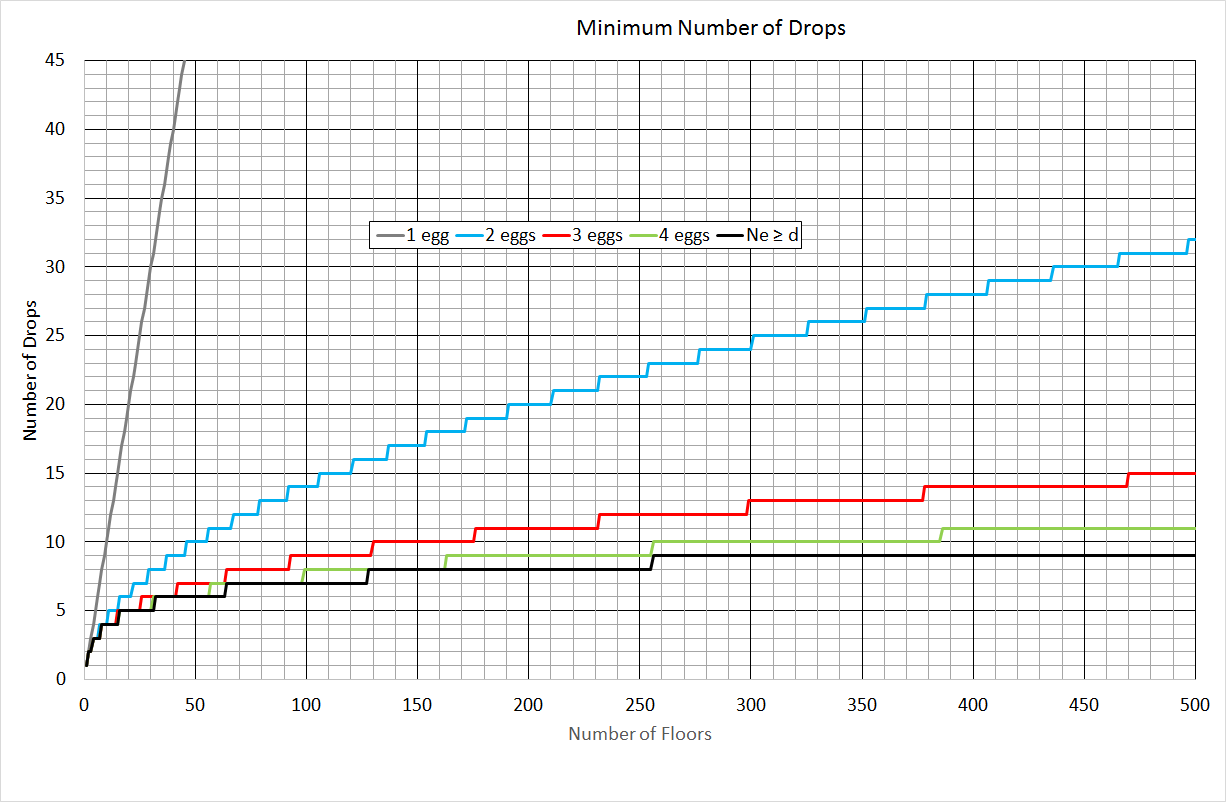You can see that two eggs is a massive improvement over one egg. Adding a third egg is pretty significant as well. With 4 eggs we have a pretty good approximation of the "many" eggs case, even in a hypothetical building with 500 floors (11 drops vs. 9 drops).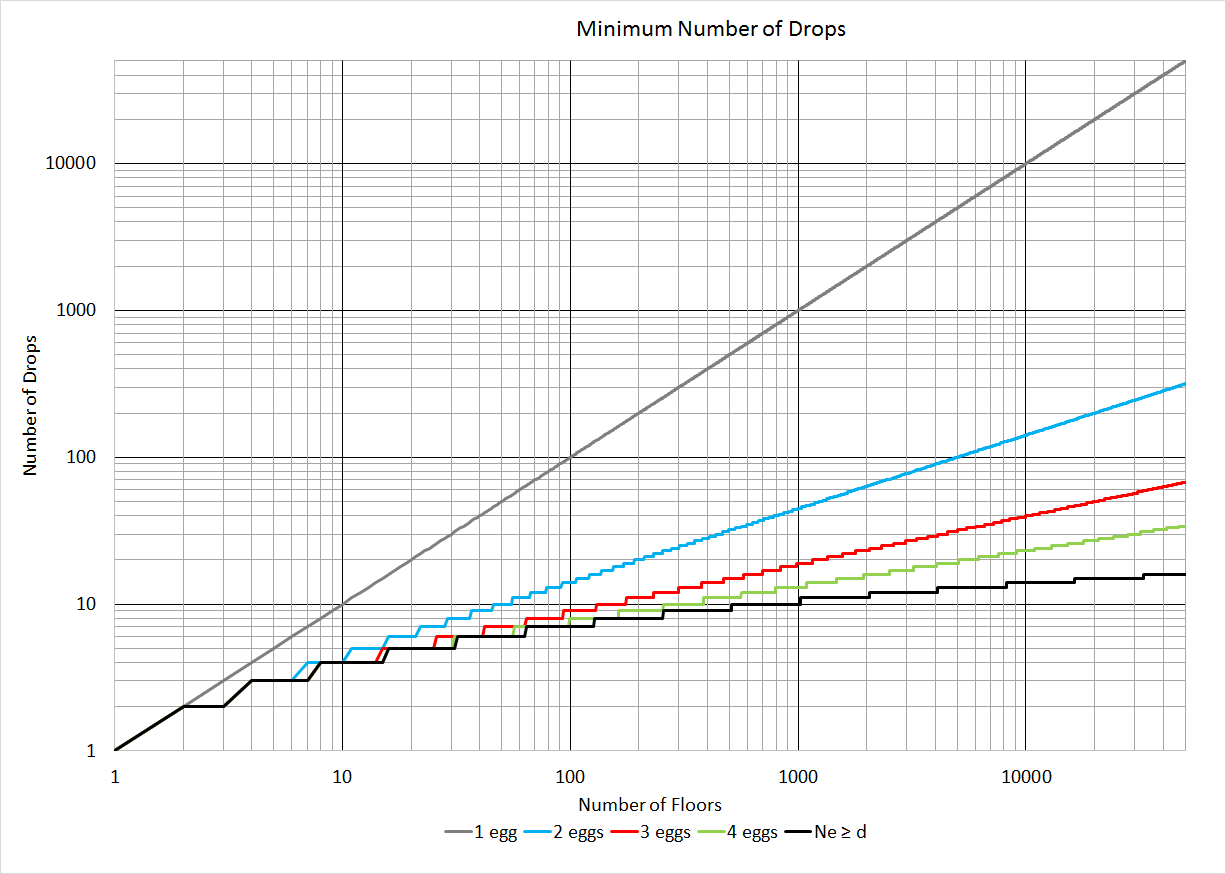In this graph I changed the axes to logarithmic scale and went all the way to 50,000 floors. I think it's a little easier to see how closely each of the D[Nf,Ne] functions approximate the best case (when Ne > d) at low values of Nf, but we also see that 4 eggs doesn't approximate "many" eggs very well beyond 500 floors.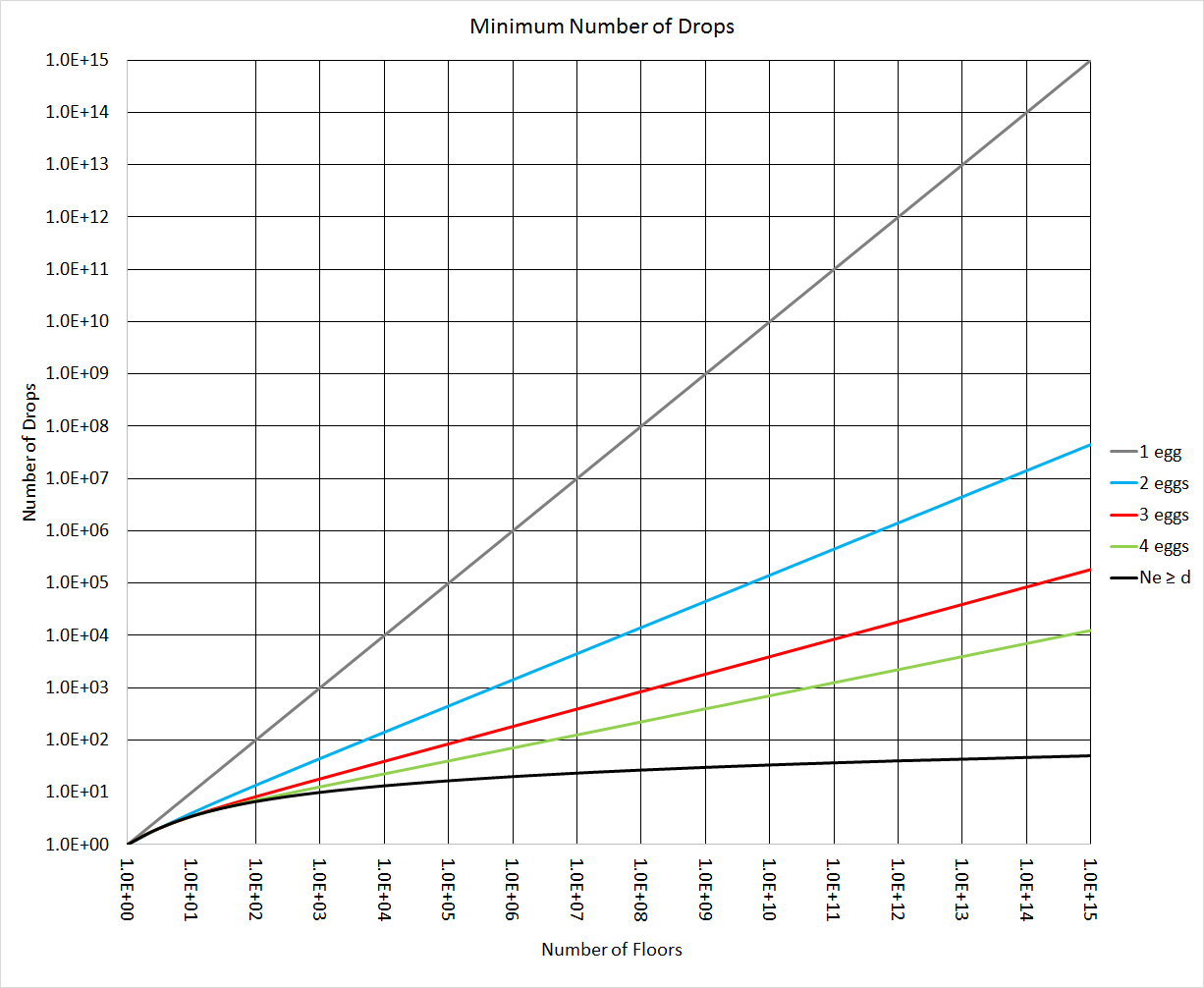In this final graph I approximated d by ignoring the steps in the function (i.e. I treated d as if it were defined for a continuum rather than just the natural numbers). That allowed me to go to an insanely high number of floors (1.0E15 = 1 quadrillion, or 1 followed by 15 zeros). At this scale you can see a significant difference between 4 eggs and "many" eggs for large values of Nf. With 30 eggs and 30 drops you could test a building with one billion floors. But if only 4 eggs were available you'd need to allow for 395 drops.